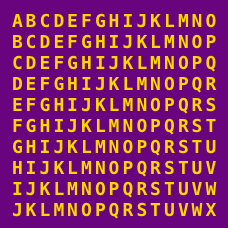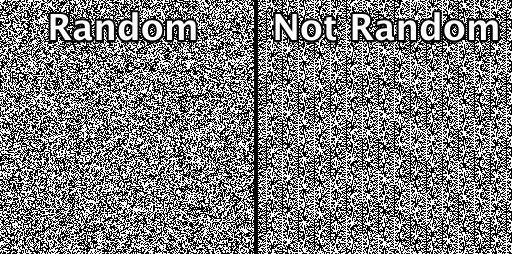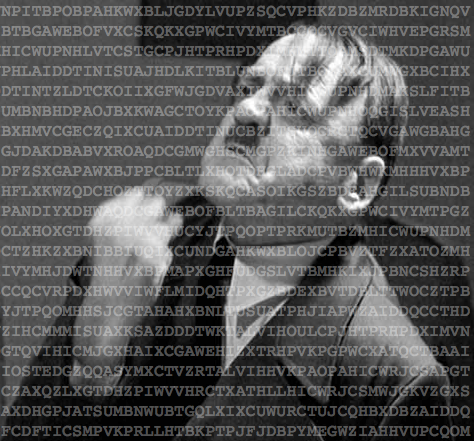Computer Science

Cryptography: Level 3 ChallengesYou've stumbled onto a significant vulnerability in a commonly used cryptographic library. It turns out that the random number generator it uses frequently produces the same primes when it is generating keys.

Exploit this knowledge to factor the (hexadecimal) keys below, and enter your answer as the last six digits of the largest factor you find (in decimal).

Key 1:
1c7bb1ae67670f7e6769b515c174414278e16c27e95b43a789099a1c7d55c717b2f0a0442a7d49503ee09552588ed9bb6eda4af738a02fb31576d78ff72b2499b347e49fef1028182f158182a0ba504902996ea161311fe62b86e6ccb02a9307d932f7fa94cde410619927677f94c571ea39c7f4105fae00415dd7d

Key 2:Here is Eddie's take on the Diffie-Hellman Cryptosystem.

However, not using large enough key sizes can be a problem.

Given below is a generator $g$, a prime $p$ and a public key $B$ such that $g^b \equiv B \pmod p$ for some private key $b$.

Find $b$

 1 2 3 g = 4567316637081665907977181518889182113445441987446007457733573698022127469439135656733423482251931597580003871195410610463070846363265764446085651841441485; p = 20992321342930071437617535054294082600409305694823917901103517516849439468932230646876254462856426279068085286595868000559162159946064999915305764788212947; B = 13261683723811565199480160483723583184154281997005060032137595421236239575828461774398757507049515905188090075911486594228158952115852574793046073896571395;It's 1944, and you have just been handed the following cipher text, intercepted from a German command post in France:

There is reason to believe that this text was not encrypted with the sophisticated German Enigma machine but instead used a Vigenère cipher with a simple pass phrase. Thus, it is vulnerable to frequency analysis.

Decode this message and find out where the Germans are sending their bombers so we can evacuate the civilians. You will enter the number of the district (as shown in this list) to submit your answer.

Details and assumptions

A Vigenère cipher is a more complicated shift cipher. For example, rather than shifting every character in a word by two letters like this:

SECRET -> UGETGV

you would instead choose a pass phrase (e.g., "CAT"), which would then tell you to shift each of the letters in your plain text by the letter in the passphrase ("C" is a shift of 2 character, "A" is a shift of none, etc. ) :

C A T C A T (passphrase)
S E C R E T (plain text)

U E V T E M (cipher text)

Note: This problem is loosely based on cryptographic methods used around the time of the invasion of Normandy in 1944.

×